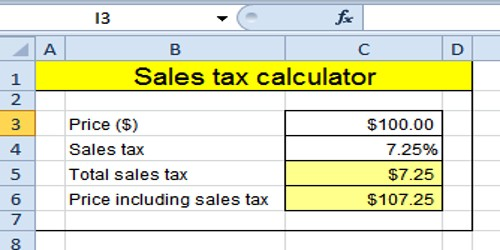Mathematic

# Calculation of Sales TaxCalculation of Sales Tax

A sales tax is the money paid to a government for the sales of certain goods and services. Usually the laws let the seller to collect the tax from the consumer when purchasing.

To calculate the sales tax that is included in a company’s receipts from items subject to sales tax, divide the receipts by 1 + the sales tax rate. For example, if the sales tax rate is 6%, divide the total amount of receipts by 1.06. If the sales tax rate is 7.25%, divide the total receipts by 1.0725. The calculation of sales tax is very easy as it involves very simple concept of percentage.

The government of every country needs money the following:

(i) to meet their administrative expenses,

(ii) to execute social welfare and development schemes,

(ii) to meet the expenses on salaries of its employees, etc.

One of the many sources of collecting money (revenue) by the government from the citizen on the sale of goods within their respective territories. This purpose is known as sales tax.

It is levied by a government on the sale of different commodity. The sales tax is the sum of money a buyer pays over and above the price of a commodity to buy it.

The rates of tax on purchase of different commodities within a country are different. Also, the rates of tax on the same commodity in different country are different. Some commodities may be be exempted from sales tax by a government. Sales tax is one of the many forms of indirect taxes that a government imposes on its citizens.

If P be the printed price or marked price of a commodity, the rate of sales tax be r% and S be the selling price (i.e., the price a customer has to pay) then

S = P (1 + r/100) and sales tax = S – P = P (1 + r/100)

Solved examples on relation between printed price, rate of sales tax and selling price:

The printed price of a bi-cycle is \$ 4200. The rate of sales tax on it is 10%. Find the price at which the cycle can be purchased.

Solution:

Here, the printed price P = \$ 4200, the rate of sales tax = 10%, i.e. r = 10

Therefore, the selling price S = P (1 + r/100)

= \$ 4200 × (1 + 10/100) = = \$ 4620

Therefore, the cycle can be purchased for \$ 4620.

Example: 2

Mason purchased a pair of shoes costing \$ 850. Calculate the total amount to be paid by him, if the rate of Sales Tax is 6%.

Solution:

The sale price of shoes = \$ 850

and, the sales tax = 6 % of \$ 850 = \$ 5

Therefore, the total amount to be paid by Rohit = \$ 850 + \$ 51 = \$ 901.

Information Source: